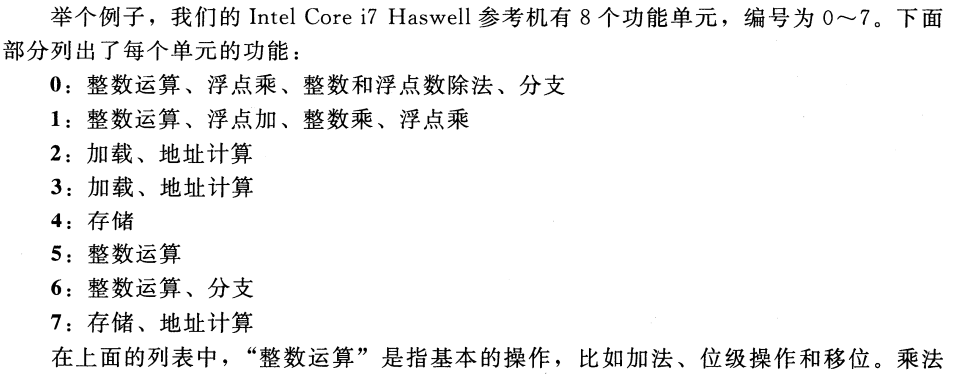# 常数优化之循环展开

## 背景## 循环展开——CPU友好型代码

### 原理

#### 流水线• $I1、I2、I3$表示待三条不同的机器指令，$A、B、C$代表指令的阶段(当然不止3个，由CPU设计者决定)

x += a;
tmp = x * b;
// 可以改为
tmp = a * b + x * b;


#### 理解现代处理器——以Intel Core i7 Haswell为例#### 循环展开

//非循环展开
for(int i = 1; i <= n; i++)
sum += a[i];

//2*1循环展开
int i;
for(i = 1; i <= n - 1; i += 2) {
sum += a[i];
sum += a[i + 1];
}
for(; i <= n; i++)
sum += a[i];

//3*1循环展开
int i;
for(i = 1; i <= n - 2; i += 3) {
sum += a[i];
sum += a[i + 1];
sum += a[i + 2];
}
for(; i <= n; i++)
sum += a[i];



2×1和3×1循环展开中的2和3很好理解，就是步长的意思，至于1是什么意思，恳请众看官耐心看下去。

• 它减少了不直接有助于程序结果的操作数的数量，如2×1展开中，i += 2的执行次数小于i++的次数，i <= n的条件判断次数也是2×1展开少
• 它提供了一些方法，可以进一步变化代码，使其性能更高

//2*2循环展开
int i, sum0, sum1, sum;
for(i = 1; i <= n - 1; i += 2) {
sum0 = sum0 + a[i];
sum1 = sum1 + a[i + 1];
}
for(i; i <= n; i++)
sum0 += a[i];
sum = sum0 + sum1;## 参考文献

posted @ 2020-02-14 10:53  Jr1Preg  阅读(...)  评论(...编辑  收藏
TOP